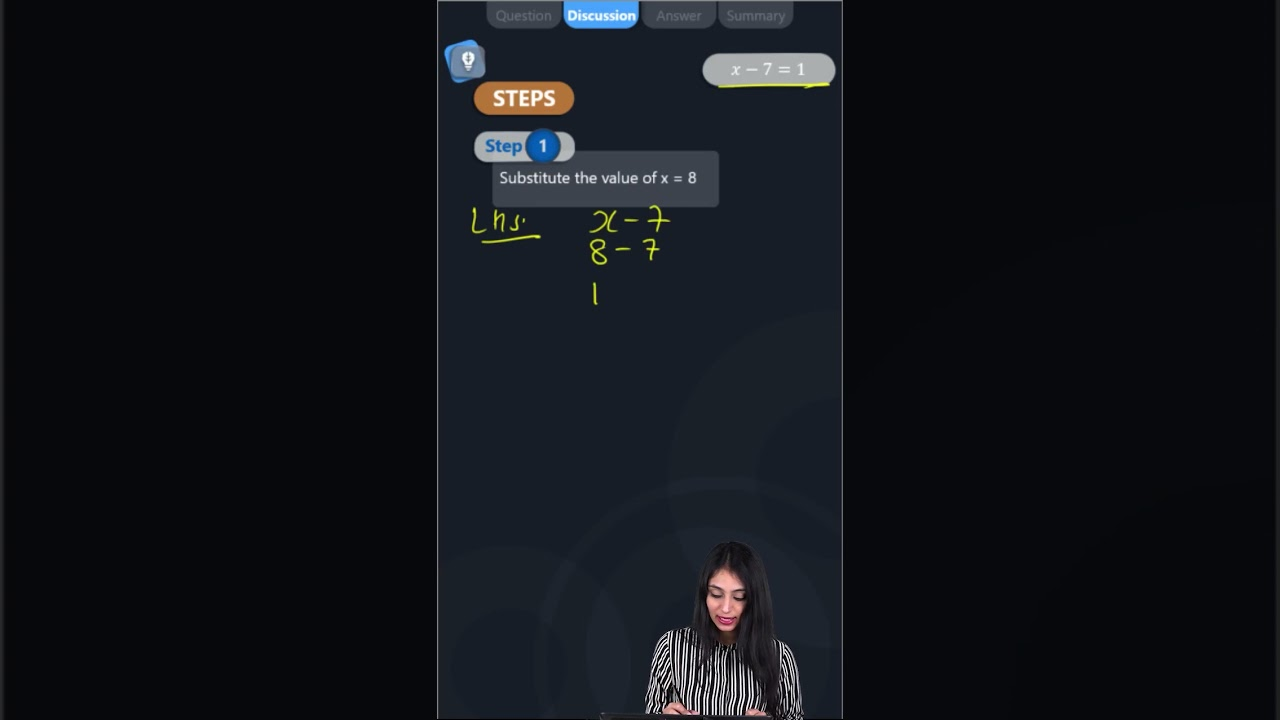Complete the last column of the table: x - 7 = 1 x = 8 Say, whether the equation is satisfied. (Yes/No)

 x – 7 = 1 x = 8 Say, whether the equation is satisfied. (Yes/No)Given: x – 7 = 1 and x = 8

Consider the LHS of the equation x – 7 = 1

LHS = x – 7

By substituting the value of x = 8

Then,

LHS = 8 – 7

LHS = 1

Consider RHS of the given equation

RHS = 1

By comparing LHS and RHS

LHS = RHS

∴ Yes, the equation is satisfied.(1)(0)